## Indicators

•Cited by SciELO
•Access statistics

•Cited by Google
•Similars in SciELO
•Similars in Google

## Print version ISSN 0120-6230On-line version ISSN 2422-2844

### Rev.fac.ing.univ. Antioquia  no.59 Medellín July/Sept. 2011

Far field from near field measurements. An approach to solve the inverse problem of electromagnetic radiation via genetic algorithms

Campo lejano a partir de mediciones de campo cercano. Una propuesta para resolver el problema inverso de radiación electromagnética con algoritmos genéticos

Arturo Rangel Merino, Roberto Linares y Miranda, José Luis López Bonilla*

ESIME-Zacatenco, Instituto Politécnico Nacional, Anexo Edificio 3, Col. Lindavista CP 07738, México D.F.

Abstract

In this paper the application ofthe Genetic Algorithms (GA) as a search method of a geometric configuration of elementary dipoles that solve the inverse problem of electromagnetic radiation are reported. The inverse problem of electromagnetic radiation appears as a new application in the electromagnetic compatibility area that allows estimating the far field radiation from near field measurements.

Keywords:Far field, near field, measurements, genetic algorithms.

Resumen

En este artículo se reporta la aplicación de los Algoritmos Genéticos como método de búsqueda de una configuración de dipolos elementales que resuelven el problema inverso de radiación electromagnética. El problema inverso de radiación electromagnética aparece como una aplicación en el área de compatibilidad electromagnética para permitir estimar las radiaciones de campo lejano a partir de mediciones de campo cercano.

Palabras clave: Campo lejano, campo cercano, mediciones, algoritmos genéticos.

Introduction

The far field electromagnetic radiation estimation is a common requirement in the electromagnetic compatibility area. For this reason, the international standards recommend limits of the emissions, which must be check in environmental controlled, such as: a normalized open area site or an anechoic chamber; it is why the evaluation of the conformity of the emission has a high costs. In this paper a new solution to estimate far fields from near field measurements in situ or controlled environments, which represents a distribution and scope of the electromagnetic real radiation, is presented. The solution is based on the inverse problem of electromagnetic radiation by means of GA, which are applied to the search of geometric elementary dipoles configurations. The problem of estimating far field when the model of radiation is known (for example, an infinitesimal dipole ) is easy, because the distance parameter of the model mentioned before is that of heavier weight. However, when we have a DR which the radiation model is unknown, the far field emission determination is carried out in the site of interest, which can be impractical, vague and expensive.

The way of overcoming these drawbacks is to take in consideration the effects of the RD in its surrounding space (near field zone), finding a dipolar configuration or structure. From this structure and applying the electromagnetic radiation inverse problem using GA, one can rebuild a radiation pattern that allows the estimation of the far field emissions behavior just by modifying the distance parameter. An optimization method is needed to resolve the problem. One can find optimization methods in the current literature, analytical as well as numerical, that are applied to electromagnetic. The analytical methods still use integrals , gradients, etc; and the numerical employs the moments method , finite differences in the time domain, furthermore, the most recent one, the simulated annealing, ants colony , genetic algorithms [5, 6] and others.

The versatility of GA [7-9] to adapt to the solution of any problem, without any mathematical condition with regards to the function that is desired to optimize, is what makes it attractive to be applied to the electromagnetic radiation inverse problem. Every problem has its own characteristics, and it is necessary to tune the AG operation parameters, as well as the ones of the same function that is desired to optimize.

Methodology

The electromagnetic radiation inverse problem is carried out by means of AG, which asks for an object function where it is important to define the problem geometry as well as the variables or parameters involved in each terms of the function. The geometry of the problematic to solve is developed from the electromagnetic fields regions, since the aim is the estimation of far field from near field measurements.

Since it is known, the equations (1) and (2) determine the field that an elementary dipole emits: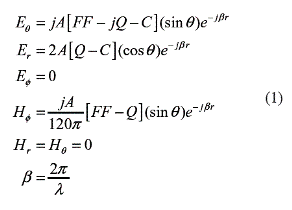where: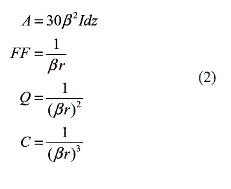From the previous equations (1) and (2) the regions of near field and far field considering the distance from RD to the observation point in electrical length have been specified, for βr = (2π/λ) r < 1 we have the near field zone. In this region it is possible to measure, and from the electromagnetic radiation inverse problem it is possible to find a geometric configuration of elementary sources to have a known model about radiation.
The measurements can be realized at any point of the zone of near surrounding field to the RD and the search of the geometric configuration can be carried out by some analytical or numerical method. As in many electromagnetic radiation problems, the RD is located at the origin of a coordinated system of global reference and the observation points or field measurement must be indexed to this system.

For solving the specific case, we propose a source structure (elementary dipoles) with random location inside the global reference system. For the location of each of the above elements mentioned before, each of them will have two orientations (θ,φ) and three position (x,y,z) parameters, besides the excitation (I0) and the phase (α) parameters, as well as, the parameter (TD) to differentiate an electrical dipole (TD=0) from a magnetic dipole (TD=1), thus the total of variables for the electromagnetic fields determination of the elementary dipoles is eight.

The total field in an observation point is calculated by the superposition theorem.

Due to the fact that the field emissions of an element of the structure dipolar is calculated based on its reference, it is necessary to realize a transformation of the observation point from the global system to the coordinates system of the dipole under study.

In order to find the radiation model parameters (the excitation, orientation and position) of every element of the configuration it is necessary to construct a function called object function. This function can conform as the difference of the measured field and the total field (superposition) of the configuration, for example, for this case the object function is, FO=Ei-(E1+E2+E3+...+En)|i, where Ei is the field measure and E1, E2, E3, ... are the field values of the dipoles 1, 2, 3, ..., n, all of them in the observation point i. If we have m points of field measures, a configuration dipolar approximate would be the one that had the lowest sum of the differences in every point. A problem like that represents an optimization problem. The object function can be parameterized by analytical or numerical methods. For this case we used the GAs, which is an optimization method that has given successful results. For its versatility and no requirements of mathematical properties of the function to optimize, the GAs are attractive to solve the electromagnetic radiation inverse problem which is the approach of this work.

Due to the previous statements and given that the field superposition is composed of harmonic own functions of the elementary dipoles field expressions, we can expect that the configuration dipolar solution to the electromagnetic radiation inverse problem is not unique. GA is based on a population of possible solutions and three basic operators, cross, mutation and selection (or reproduction according to Goldberg ). These operators applied successively to the population (evolution) can produce an individual with wanted characteristics and these could be reflected in the object function. Each application of the operators to the population is called generation. The population is constructed from a non fixed quantity of individuals, generally it is a matricial arrangement, the rows of such arrangement are individuals formed from the variable quantities on which the problem depends, that is to say, an individual can be formed as a numerical arrangement with as many elements as variables the problem have.

The initial population, needed for GA, is constructed generally as a random matrix that respects the space values for every variable. Cross is an operator whose function is to exchange the variables (genes or row entries) of two individuals, the above mentioned exchange can be carried out of fixed form (always in the same position), random form (position does not fix) or under some scheme designed for it. The mutation is an operator whose task is to change randomly some genes of one or more individuals of the population. The selection is a function that selects the individuals who have better response to the object function value expected.

Results and discussion

The availability of optimization programs based on GAs is vast, and any chosen package must be studied and tested before being applied to the problem in question.

For the problem to solve, in this document the results were obtained using MatLab  employing one GA real valued. The method was applied to a typical electromagnetic compatibility problem on electronic ballasts lights. The evaluation of the conformity of the electronic ballasts for the radiated emissions is carried out in anechoic chambers or normalized sites, nevertheless, in its real environment of operation it is not possible to affirm that they do not produce disturbances to equipments or systems operating in its vicinity, so it is important to determine the behavior and shape of the radiation that they emit. The radiation pattern, of electronic ballast for discharge lamps of high intensity, measured in an anechoic chamber is sketched in the figure 1).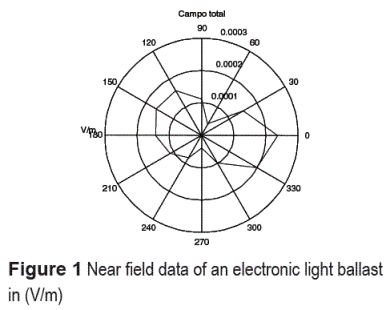With the near field data and a radiation model we apply the AG. The radiation models obtained are depicted in the tables 1 and 2, and the corresponding pattern are showed in the figures 2 and 3.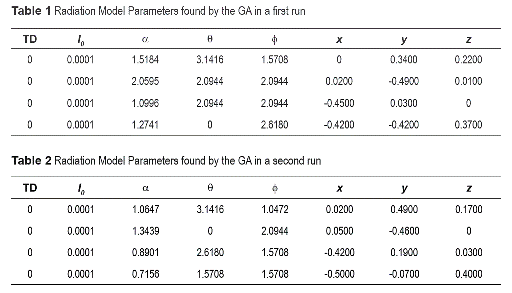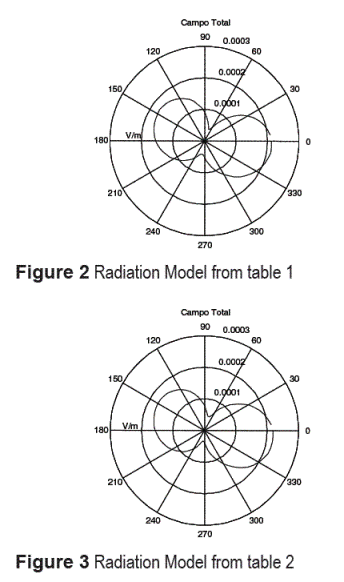With the obtained radiation models it is possible to predict the far field behavior only modifying the distance parameter.

Conclusion

The approach of a radiation model parametrized via GA in regard to the emission of a RD (ballast) is valid for electromagnetic compatibility problems, since it allows estimation of the coverage of the radiated emission in far field zone. The GA that carries out the optimization does not need complicated schemes on the selection operator and it is sufficient with only three operators and a population to apply the above mentioned operators. Nevertheless, it is very important that the GA respects the quest interval for every variable of the object function at all times, given that the GA process functions with random nature operators and the time consumption can become excessive. The time of the process may swoop down if GA can incorporate a programmable resolution for every variable. By the information in the tables 1 and 2 it is confirmed that the solution to the inverse problem of the electromagnetic radiation is not unique, in this case those of major weighting are reported, and in them it is possible to observe the differences in the parameters of every radiation model.

In spite of the differences of parameters values in the above tables, the radiation pattern plotted by both models are almost identical, this confirms that the solution is not unique. The results presented in this work correspond to the optimization of a object function of one aim, for which the case of the analyzed ballast (measurements) are to the electrical total field, which does emphasis in the contribution of this work, and is the approach of a solution of the inverse problem of electromagnetic radiation.

References

1. C. A. Balanis. Antenna Theory Analysis and Design. 2a. ed. Ed. Wiley. New York. 1987. pp. 134-207.         [ Links ]

2. R. Laroussi, G. Costache. "Far-Field Predictions from Near-Field Measurements Using an Exact Integral Equation Solution". IEEE Trans on EMC. Vol. 36. 1994. pp. 189-195.         [ Links ]

3. W. D. Rawle. "The Method of Moments: A numerical Technique for Wire Antenna Design". High Frequency Electronics. Vol. 5. 2006. pp. 42-47.         [ Links ]

4. C. M. Coleman, J. Rothwell, J. E. Ross. "Investigation of Simulated Annealing, Ant-Colony Optimization and Genetic Algorithms for Self-Structuring Antenna". IEEE Trans on Antennas and Propagation. Vol. 52. pp. 1007-1014.         [ Links ]

5. T. S. Sijher, A. A. Kishk. "Antenna Modeling by Infinitesimal Dipoles Using Genetics Algorithms". Progress in Electromagnetics Research PIER. Vol. 52 . p. 225-254.         [ Links ]

6. B. Liu, L. Beghou, L. Pichon. "Adaptive Genetic Algorithm Based Source Identification with Near-Field Scanning". Progress in Electromagnetics Research B. Vol. 9. 2008. pp. 215-230.         [ Links ]

7. D. E. Goldberg. Genetic Algorithms. Ed. Addison Wesley. New York. 1989. pp. 126-129.         [ Links ]

8. R. L. Haupt. "An Introduction to Genetic Algorithms for Electromagnetics". IEEE Antennas and Propagation Magazine. Vol. 37. 1995. pp. 7-15.         [ Links ]

9. A. Rangel Merino, J. L. López Bonilla Linares, R. Miranda. "Optimization Method based on Genetic Algorithms". Apeiron. Vol. 12. 2005. pp. 393-408.         [ Links ]

10. Matlab 7.0. R14. 2004.All the contents of this journal, except where otherwise noted, is licensed under a Creative Commons Attribution License